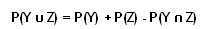﻿
A statistical property that states the probability of one and/or two events occurring at the same time is equal to the probability of the first event occurring, plus the probability of the second event occurring, minus the probability that both events occur at the same time.

Mathematically, this property is denoted by:For example, assume we wish to determine the probability of drawing a king and/or a queen out of a deck of cards on only one draw. Using the addition rule for probabilities, we get the following: P(King) = 4/52, P(Queen) = 4/52, and P(King and Queen) = 0.

Since it is impossible to draw both a king and queen on the same draw, we can conclude that the probability of drawing either a king or queen from a deck of cards is 8/52, or about 15.4%.

Investment dictionary. . 2012.

### Look at other dictionaries:

• Mathematics and Physical Sciences — ▪ 2003 Introduction Mathematics       Mathematics in 2002 was marked by two discoveries in number theory. The first may have practical implications; the second satisfied a 150 year old curiosity.       Computer scientist Manindra Agrawal of the… …   Universalium

• DNA profiling — Not to be confused with Full genome sequencing. Forensic science …   Wikipedia

• Dempster–Shafer theory — Prof Arthur P. Dempster at the workshop on Belief Function Theory (Brest 1 april 2010). The Dempster–Shafer theory (DST) is a mathematical theory of evidence. It allows …   Wikipedia

• probability theory — Math., Statistics. the theory of analyzing and making statements concerning the probability of the occurrence of uncertain events. Cf. probability (def. 4). [1830 40] * * * Branch of mathematics that deals with analysis of random events.… …   Universalium

• The Nature of Rationality — is an exploration of practical rationality written by Robert Nozick and published in 1993. It views human rationality as an evolutionary adaptation. Its delimited purpose and function may be responsible for biases and blind spots, possibly… …   Wikipedia

• radiation — radiational, adj. /ray dee ay sheuhn/, n. 1. Physics. a. the process in which energy is emitted as particles or waves. b. the complete process in which energy is emitted by one body, transmitted through an intervening medium or space, and… …   Universalium

• mathematics — /math euh mat iks/, n. 1. (used with a sing. v.) the systematic treatment of magnitude, relationships between figures and forms, and relations between quantities expressed symbolically. 2. (used with a sing. or pl. v.) mathematical procedures,… …   Universalium

• Charles Peirce — Infobox Scientist name = Charles Peirce box width = image size = 200px caption = Charles Peirce birth date = September 10, 1839 birth place = Cambridge, Massachusetts death date = April 19, 1914 death place = residence = citizenship = nationality …   Wikipedia

• Decision theory — in economics, psychology, philosophy, mathematics, and statistics is concerned with identifying the values, uncertainties and other issues relevant in a given decision, its rationality, and the resulting optimal decision. It is closely related to …   Wikipedia

• basketball — /bas kit bawl , bah skit /, n. 1. a game played by two teams of usually five players each on a rectangular court having a raised basket or goal at each end, points being scored by tossing the ball through the opponent s basket. 2. the round,… …   Universalium